# The Algorithm

## Briefly...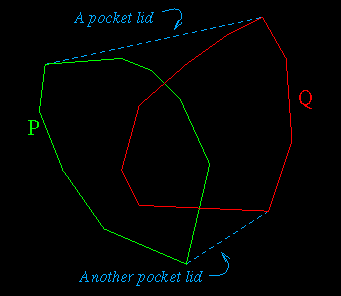Let P and Q be two convex polygons whose intersection is a convex polygon.The algorithm for finding this convex  intersection polygon can be described by these three steps:
1. Construct the convex hull of the union of P and Q;
2. For each pocket lid of the convex hull, find the intersection of P and Q that lies in the pocket;
3. Merge together the polygonal chains between the intersection points found in 2.
What's a pocket lid?
A pocket lid is a line segment belonging to the convex hull of the union of P and Q, but which belongs to neither P nor Q.

Why does it connect a vertex of P with a vertex of Q?
A pocket lid connects a vertex of P with a vertex of Q; if it were to connect two vertices of P, then P would not be convex, since the lid lies on the convex hull and is not a segment of P.

## In detail...

Computing the Convex Hull: the Rotating Calipers

To compute the convex hull of the two convex polygons, the algorithm uses the rotating calipers. It works as follows:

1. Find the leftmost vertex of each polygon.
2. At each of those two vertices, place a vertical line passing through it. Associate that line to the polygon to which the vertex belongs. The line does not intersect its associated polygon, since the polygon is convex . See the figure below:

3.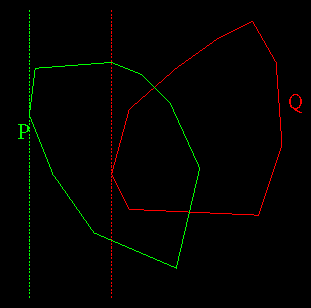4. Rotate these two lines (called calipers) by the smallest angle between a caliper and the segment following the vertex it passes through (in clockwise order). The rotation is done about the vertex through which the line passes on the associated polygon. If the line passes through more than one vertex of the assciated polygon, the farthest (in clockwise order) is taken. The result is show below:

5.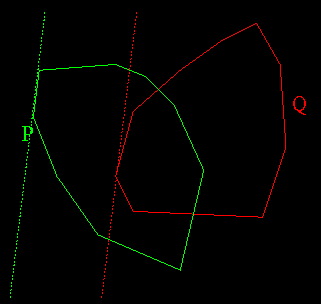6. Whenever the order of the two calipers change, a pocket has been found. To detect this, a direction is associated to one of the lines (for example the green one, associated to P). Then all points of the red line (associated to Q) are either to the left or to the right of the green line. When a rotation makes them change from one side to the other of the green line, then the order of the two lines has changed. Here's what our example looks like just before and after the algorithm has found the first pocket; as you can see, if the line associated with P initially had its associated direction pointing up, then the line associated with Q was to the right of it at the beginning, and is now to the left of it:

7.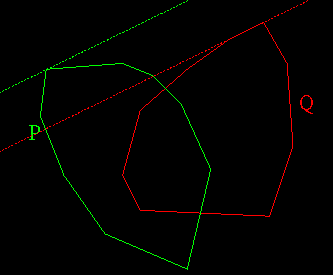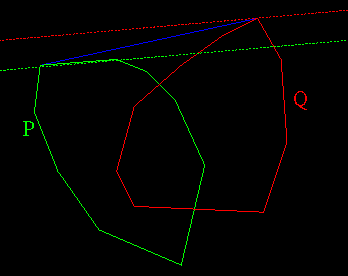8. The algorithm terminates once it has gone around both polygons.
Finding the intersection of P and Q in the pocket

Once the pockets have been found, the intersection of the polygons at the bottom of the pocket needs to be determined. The pockets themselves form a very special type of polygon: a sail polygon: that is, a polygon composed of two concave chains sharing a common vertex at one extermity, and connected by a segment (the mast) at the other end. By a procedure similar to a special-purpose triangulation for sail polygons, the segments of P and Q which intersect can be identified  in O(k+l), where k and l are the number of vertices of P and Q which are inside the pocket. The idea is to start the triangulation from the mast, and as points from P and Q are considered, a check is made to see that the chain from Q is still on the same side as the chain from P.

Here is a pseudo-code of this algorithm. It is assumed that the indices of the vertices of P and Q are in increasing order from the lid to the bottom of the pocket (i.e.: P and Q are not enumerated in the same order).

Procedure FindBottom

i <- 1; j <- 1;

repeat
finished <- true;
while ( leftTurn( p(i), p(i+1), q(j+1) ) ) do
begin
j <- j + 1;
finished <- false;
end
while ( rightTurn( q(j), q(j+1), p(i+1) ) ) do
begin
i <- i + 1;
finished <- false;
end
until finished

At the end of this procedure, the indices i and j indicate the vertices of P and Q, respectively, which are at the start of the two intersecting segments (in other words, the two intersecting segments are  p(i),p(i+1)  and   q(i),q(i+1). The intersection of these two segments is part of the intersection polygon, and can be found with your favorite line intersection formula.

Merging

What remains to be done is to build the resulting polygon. One way of doing this is to start at one of the vertices given by the above algorithm,  compute  the intersection, add that point, and then to continue adding points by following either P or Q deeper below the pocket until it comes out of another pocket (i.e. until the vertex to consider for addition happens to have been the output of the algorithm for another pocket). Then from that pocket the chain of the other polygon can be followed under the pocket. This would be done until  the pocket the chain comes out of is the pocket that the merging started with.

Checking for intersection

All of this assumes that the polygons do intersect. However, there are three ways in which no polygonal intersection could exist:

1. The intersection is either a point or a line. No provisions are made for this in the algorithm, and in this case the output will be a polygon consisting of either two vertices at the same location(in the case of a point), or  four vertices on two distinct locations (in the case of a line);
2. The polygons simply do not intersect each other and are seperable;
3. The polygons are one inside another. One could argue that the intersection of two such polygons is the contained polygon, but that is the computer graphics way of seeing things. In mathematics, there is no intersection in such a case. In any event, the algorithm has to detect this case independently of wether or not it outputs it as an intersection or not.
For case 2, this is detected if, during the triangulation step, the algorithm makes a complete loop around one of the polygons. Detecting case 3 is even easier to detect ; in such a case no pockets will be found by the convex hull algorithm.

## Implementation notes

As implemented in the applet, the algorithm will only find intersections which form non-degenerate polygons.  In other words, it will not handle properly intersections which consist of a single line or point.  However, the algorithm does detect the cases where no intersection exists at all: in the case where one polygon is contained in another, the rotating calipers will not find any pockets; in the case where the two polygons are completely outside one another, the triangulation algorithm will detect that it has looped around one of the polygons.
Also, the assumption of generality of the point positions also holds in the implementation. If the points are not in general position,  the intersection might be a degenerate polygon, or the intersection polygon might contain two consecutive segments which lie on the same line.
In the article, one polygon is enumerated in clockwise order, the other in counter-clockwise order. The implementation has both polygons in clockwise order; it simply involves a bit more housekeeping  and a bit of extra care in the merging step.

Forcing input of a convex polygon

The interface for building the polygons has the nice feature (or annoying feature, depending on your character),  of forcing the user to enter a convex polygon in clockwise order. This is done with a simple online algorithm that checks the following conditions while inserting a new vertex  i+1 between vertex i and vertex i+2:

• vertices i-1, i, i+1 form a right turn;
• vertices i, i+1, i+2 form a right turn;
• vertices i+1,  i+2, i+3 form a right turn.
In the code, this is actually implemented as a point being to the left (over) or to the right (under) of a line, rather than left turns and right turns, but the idea is exactly the same.